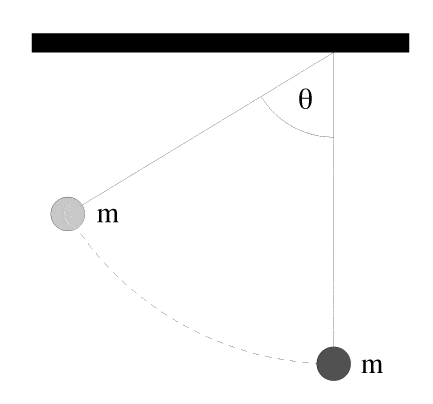# Steel Ball Collision problem

bulldog23
[SOLVED] collision problem

## Homework Statement

Two identical steel balls, each of mass 3.4 kg, are suspended from strings of length 27 cm so that they touch when in their equilibrium position. We pull one of the balls back until its string makes an angle theta = 74o with the vertical and let it go. It collides elastically with the other ball.
A) How high will the other ball rise above its starting point?
B)Suppose that instead of steel balls we use putty balls. They will collide inelastically and remain stuck together after the collision. How high will the balls rise after the collision?P=m*v
m1v1=m2v2

## The Attempt at a Solution

I am unsure how to start this problem. I know that the total initial momentum must equal the total final momentum, because the collision is elastic. Can someone help me out by explaining part A and part B?

saket
I know that the total initial momentum must equal the total final momentum, because the collision is elastic.

I think, the reason u are giving for your assertion is, simply put, WRONG!
Initial momentum = Final Momentum, if net force in the direction of the momentum is zero!
Collison is elastic => No energy loss.

In the part (A).. as collison is elastic, u can apply conservation of energy. (Of course, u have to apply once conservation of linear momentum also.)

Doing part (A), must give u enough hints to do the second part.

bulldog23
can you explain part A more. How do I apply conservation of energy and conservation of momentum?

bulldog23
How do I find the height of the first ball?

robbondo
use your trig skills... so angle is theta, the height would be possibly the adjacent side? You are give the hypotenuse. I think u can take it from there.

saket
Initially, height of first ball is R[1 - Cos(theta)], where R is the length of the string and theta is the angle mentioned in problem.
Find speed of first ball when it is just about to strike second ball.
When first ball strikes second ball, it has speed in horizontal direction. Apply collision principles to find final speed just after collision of the two balls. And, then get the height attained by each of the balls.
Note that, tension is always perpendicular to the displacement of either ball, so work done by it will be zero!

bulldog23
so I set 1/2mv^2 equal to mgh, and solved for the velocity. I got 1.95 m/s. Am I doing this right?

saket
solve the problem totally.. and then seek help. if u ask after every step... it's like being dependent on us and not beieving urself.

bulldog23
alright so I did do it right. The initial height of ball 1 equals the final height of ball 2 because the collision is elastic. So how do I approach part B?

saket
do it exactly the same way. just be careful, while applying principles of collision. Here, after the collision the two balls are going to stick together. Take this into account for conservation of linear momentum, and u will be able to do it.

bulldog23
I tried using 1/2(m1+m2)v2=(m1+m2)gy. I tried solving for y, but I am not getting the right answer. Can you explain where I am going wrong?

the time

What characteristic of Momentum makes it SO useful to engineers and scientists when analyzing explosions and collisions?Before something explodes the object is often not moving but after it explodes pieces are moving in all directions with varying speeds. How
can it be said that the net momentum after the explosion is zero?